# ggplot2: Quick Tour

### Introduction

This is the second post in the series Elegant Data Visualization with ggplot2. In the previous post, we understood the concept of grammar of graphics and even built a bar plot step by step while exploring the different components of a plot/chart. In this post, we will learn to quickly build a set of plots that are routinely used to explore data using `qplot()`. It can be used to quickly create plots but also has certain limitations. Nevertheless, if you want to quickly explore data using a single function, `qplot()` is your friend.

### Libraries, Code & Data

We will use the following libraries in this post:

All the data sets used in this post can be found here and code can be downloaded from here.

#### Scatter Plot

Scatter plots are used to examine the relationship between two continuous variables. The relationship can be examined across the levels of a categorical variable as well. Let us begin by creating scatter plots. The first two inputs are the variables/columns representing the X and Y axis. The next input is the name of the data set.

``qplot(disp, mpg, data = mtcars)``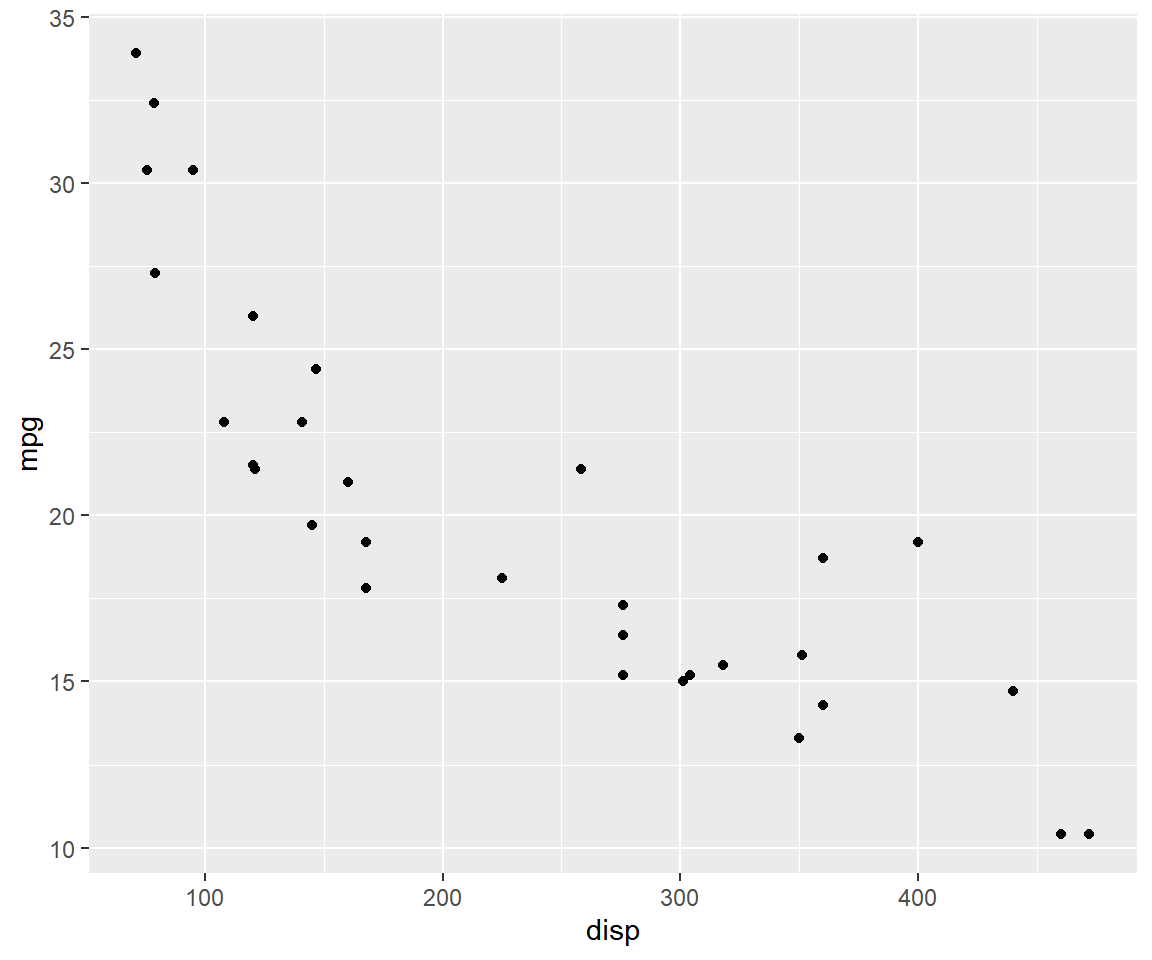If you want the relationship between the two variables to be represented by both points and line, use the `geom` argument and supply it the values using a character vector.

``qplot(disp, mpg, data = mtcars, geom = c('point', 'line'))``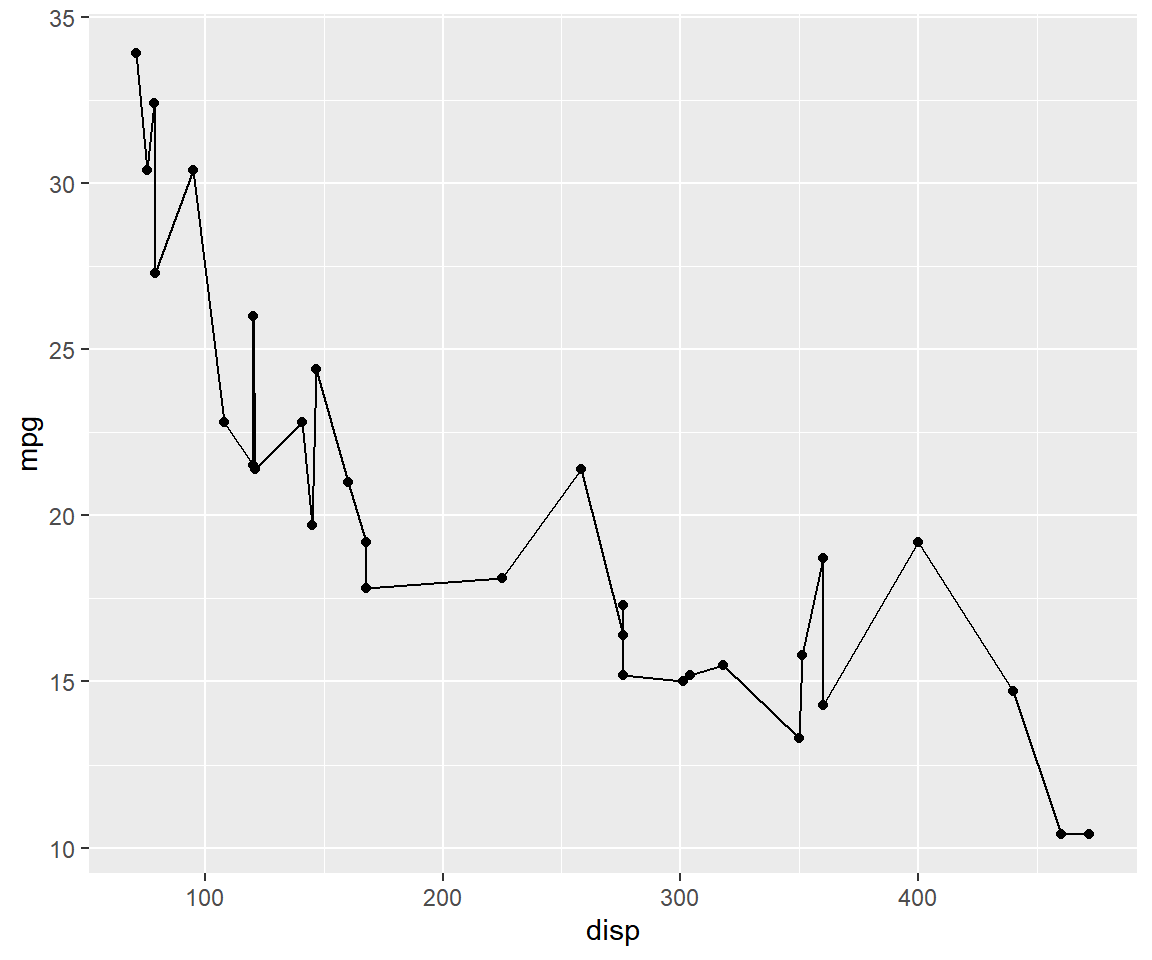The color of the points can be mapped to a categorical variable, in our case `cyl`, using the color argument. Ensure that the variable is categorical using `factor()`.

``qplot(disp, mpg, data = mtcars, color = factor(cyl))``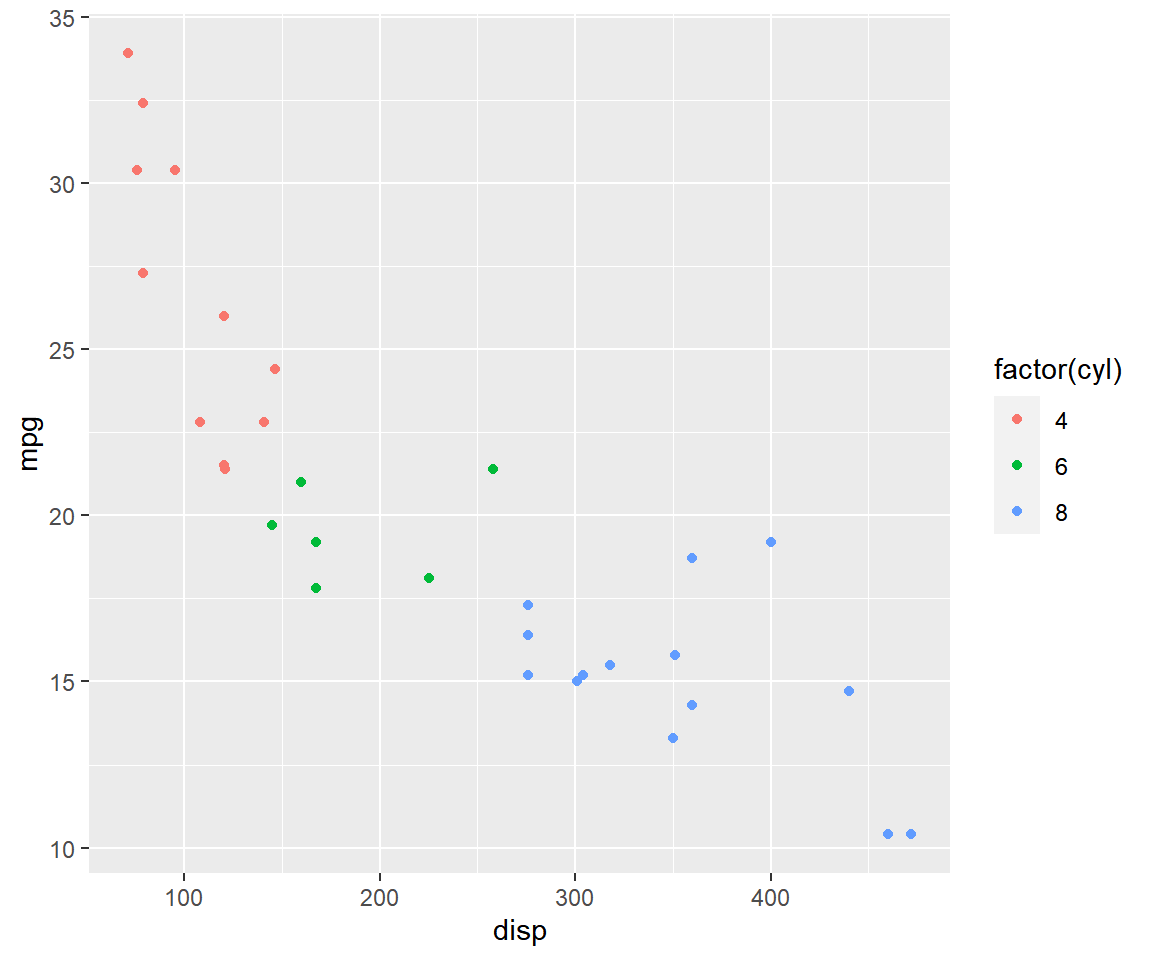The shape and size of the points can also be mapped to variables using the `shape` and `size` argument as shown in the below examples.

``qplot(disp, mpg, data = mtcars, shape = factor(cyl))``Ensure that size is mapped to a continuous variable.

``qplot(disp, mpg, data = mtcars, size = qsec)``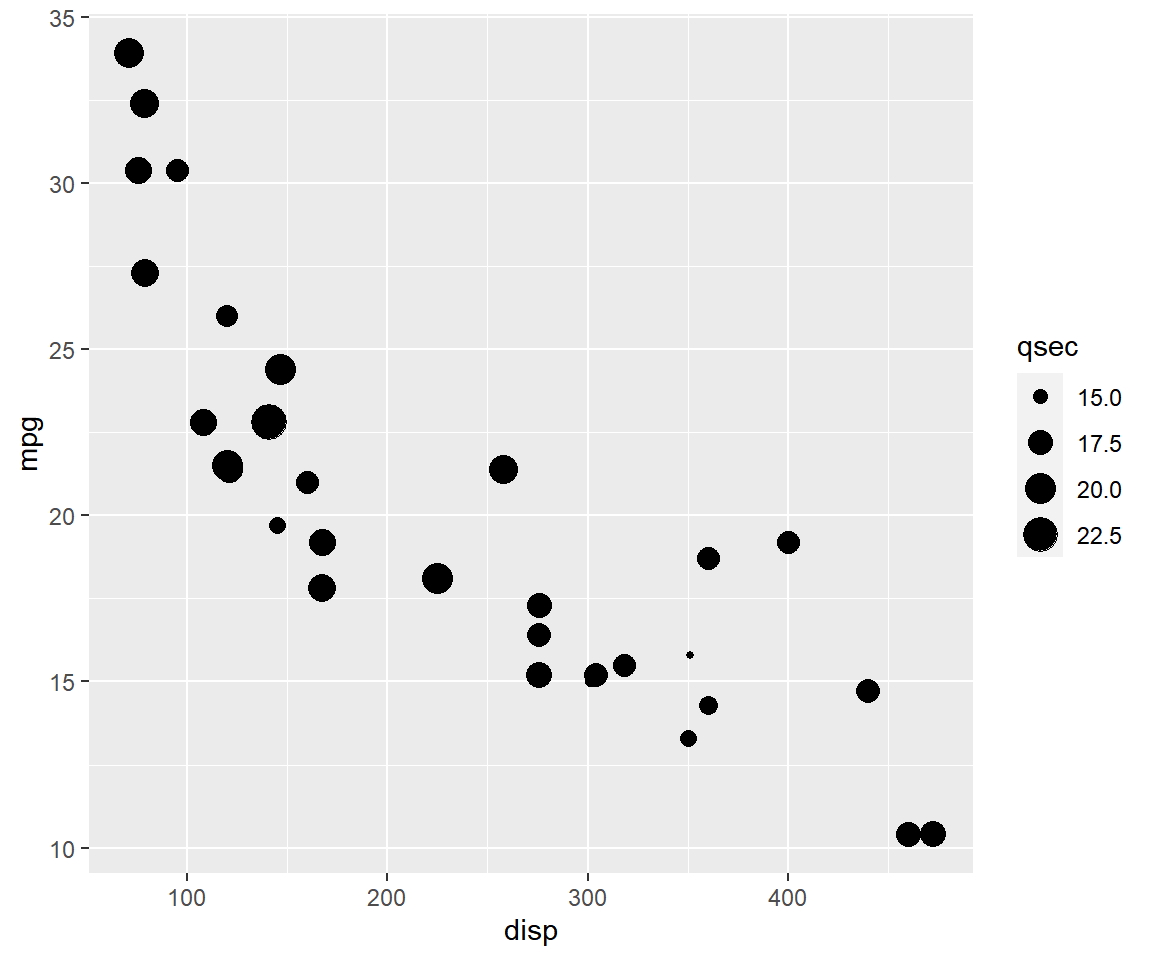#### Bar Plot

A bar plot represents data in rectangular bars. The length of the bars are proportional to the values they represent. Bar plots can be either horizontal or vertical. The X axis of the plot represents the levels or the categories and the Y axis represents the frequency/count of the variable.

To create a bar plot, the first input must be a categorical variable. You can convert a variable to type `factor` (R equivalent of categorical) using the `factor()` function. The next input is the name of the data set and the final input is the `geom` which is supplied the value `'bar'`.

``qplot(factor(cyl), data = mtcars, geom = c('bar'))``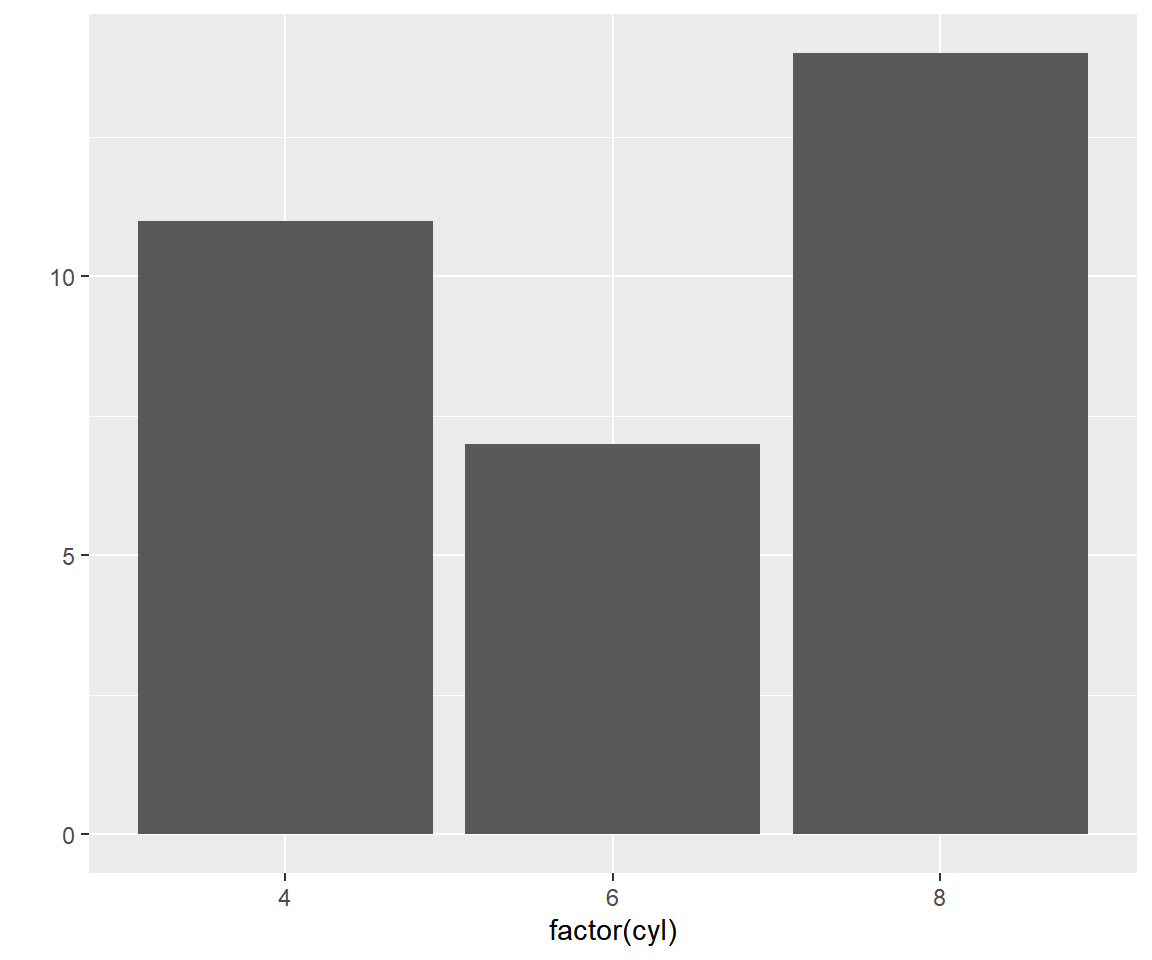You can create a stacked bar plot using the `fill` argument and mapping it to another categorical variable.

``qplot(factor(cyl), data = mtcars, geom = c('bar'), fill = factor(am))``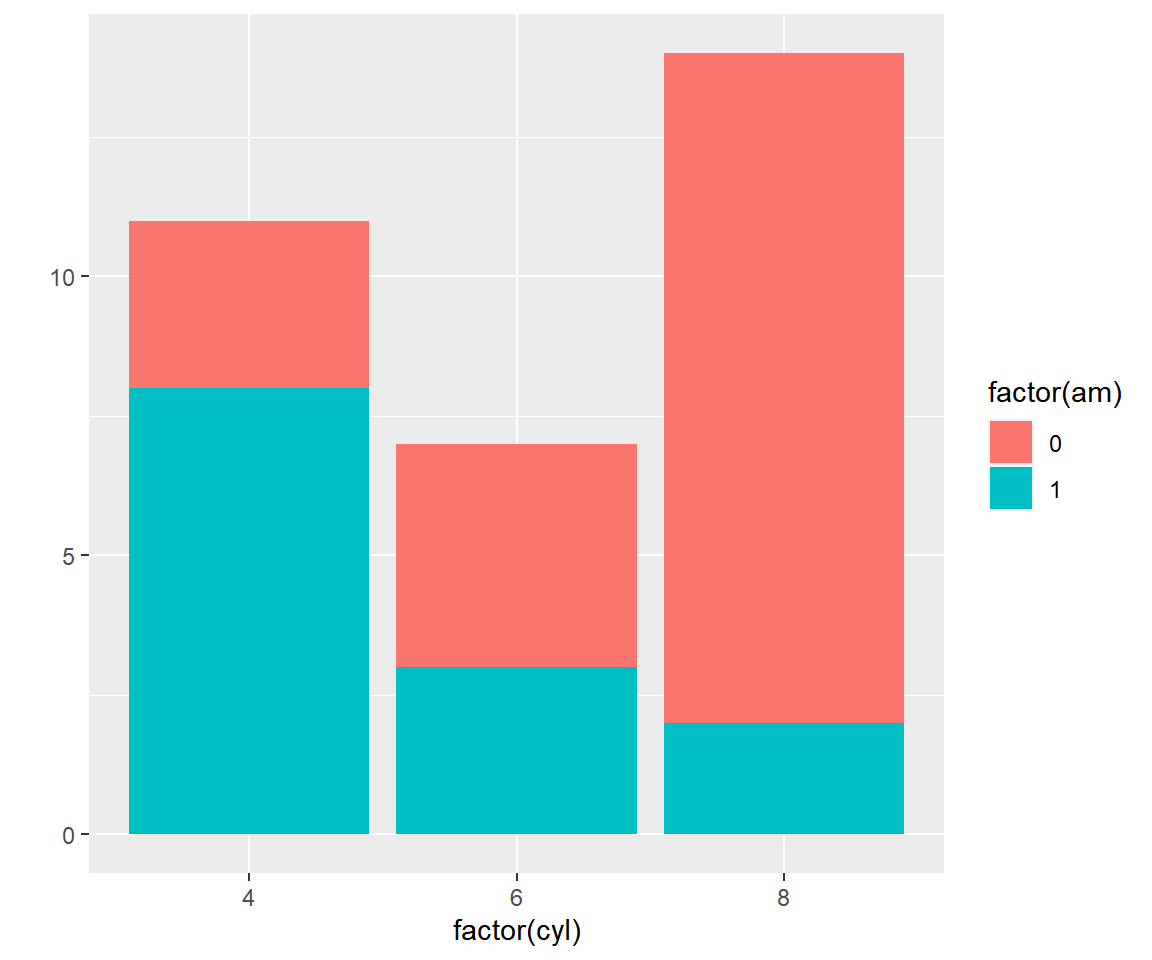#### Box Plot

The box plot is a standardized way of displaying the distribution of data based on the five number summary: minimum, first quartile, median, third quartile, and maximum. Box plots are useful for detecting outliers and for comparing distributions. It shows the shape, central tendancy and variability of the data.

Box plots can be created by supplying the value `'boxplot'` to the `geom` argument. The firstinput must be a categorical variable and the second must be a continuous variable.

``qplot(factor(cyl), mpg, data = mtcars, geom = c('boxplot'))``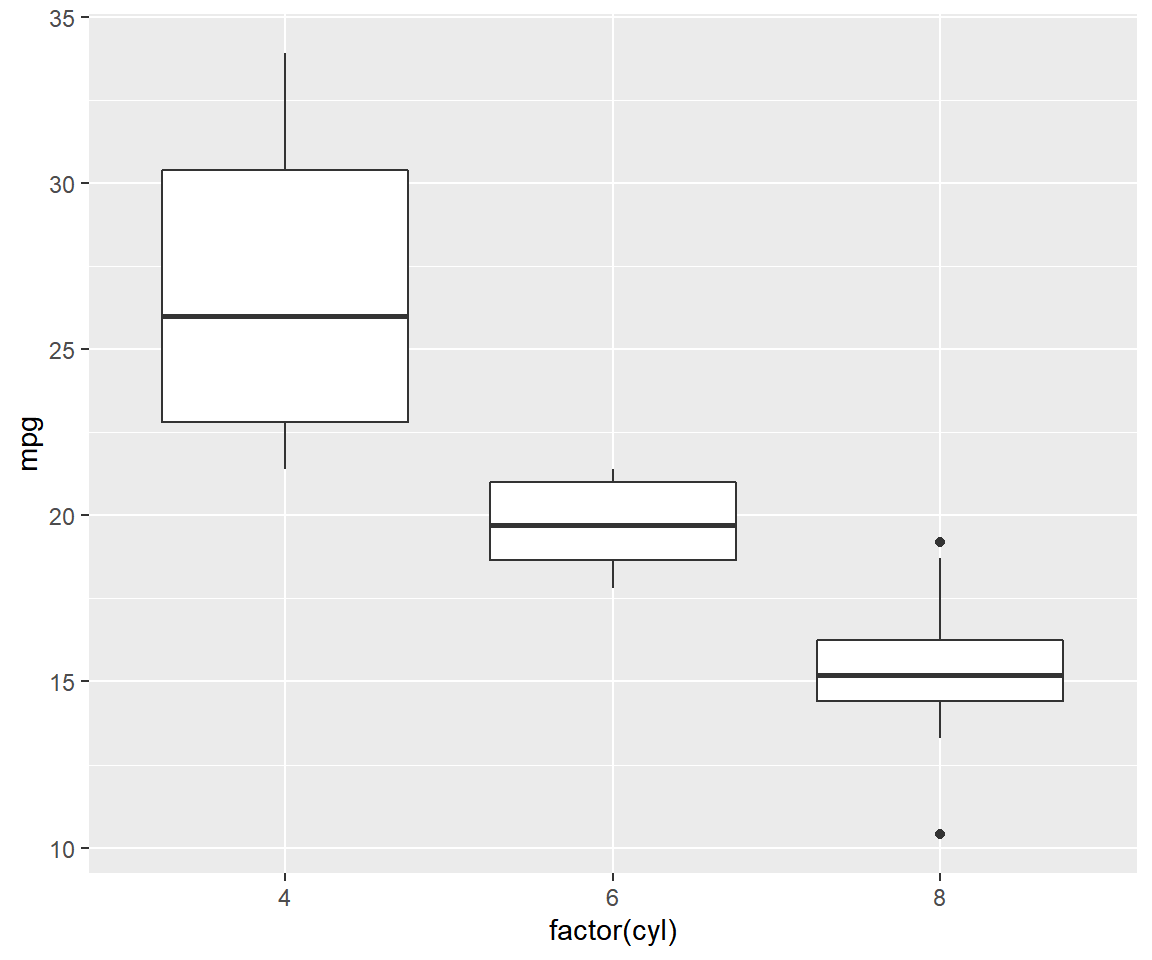Unlike `plot()`, we cannot create box plots using a single variable. If you are not comparing the distribution of a variable across the levels of a categorical variable, you must supply the value `1` as the first input as show below.

``qplot(factor(1), mpg, data = mtcars, geom = c('boxplot'))``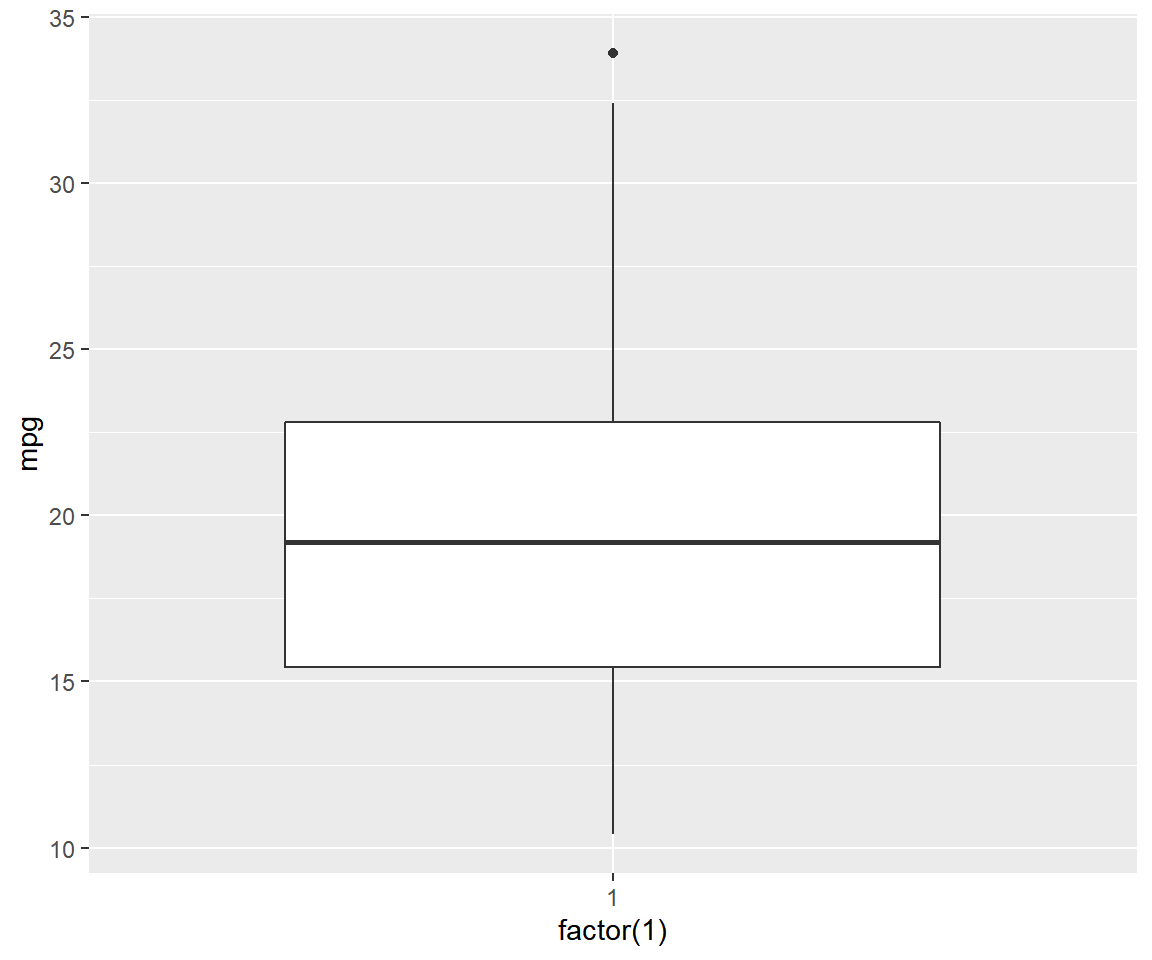#### Line Chart

Line charts are used to examing trends across time. To create a line chart, supply the value `'line'` to the `geom` argument. The first two inputs should be names of the columns/variables representing the X and Y axis, and the third input must be the name of the data set.

``qplot(x = date, y = unemploy, data = economics, geom = c('line'))``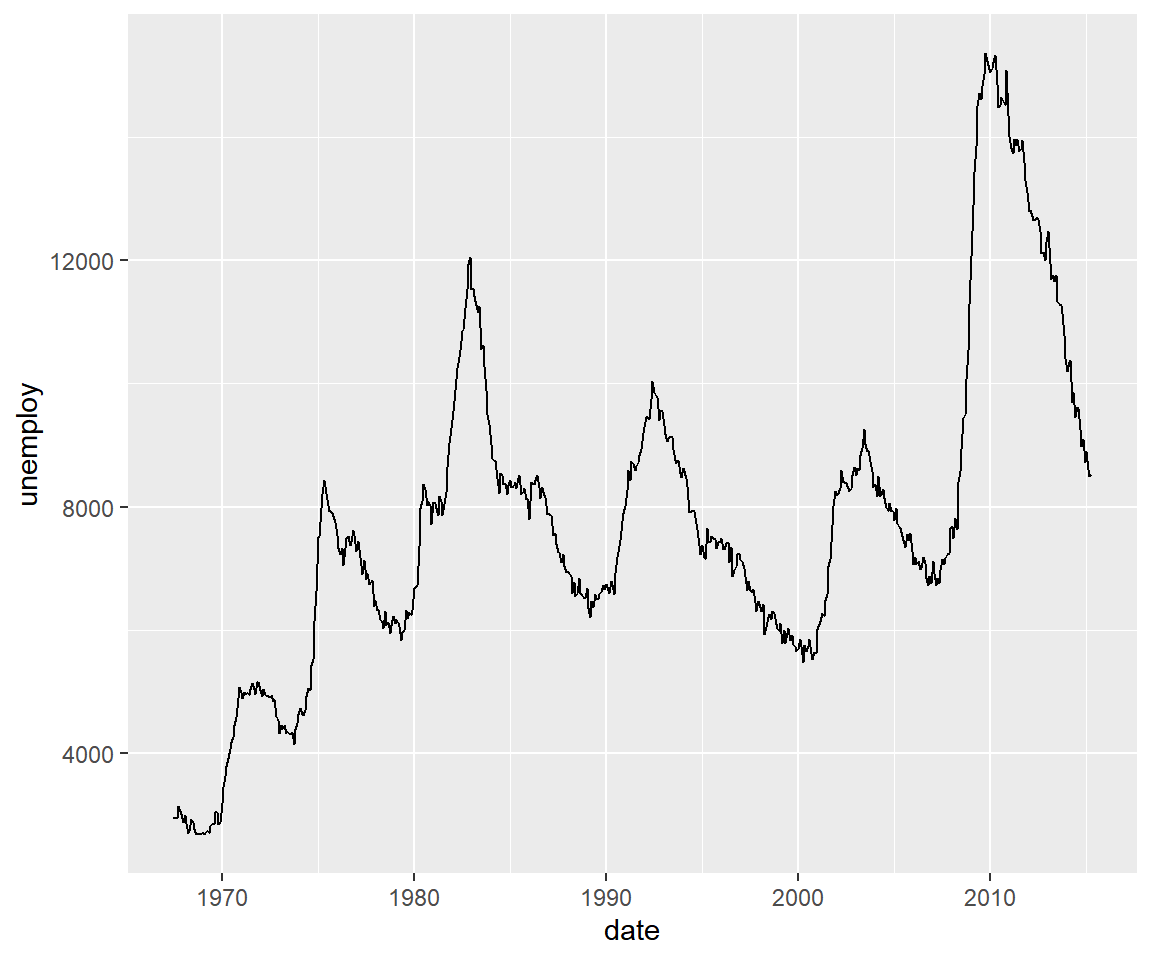The appearance of the line can be modified using the `color` argument as shown below.

``````qplot(x = date, y = unemploy, data = economics, geom = c('line'),
color = 'red')``````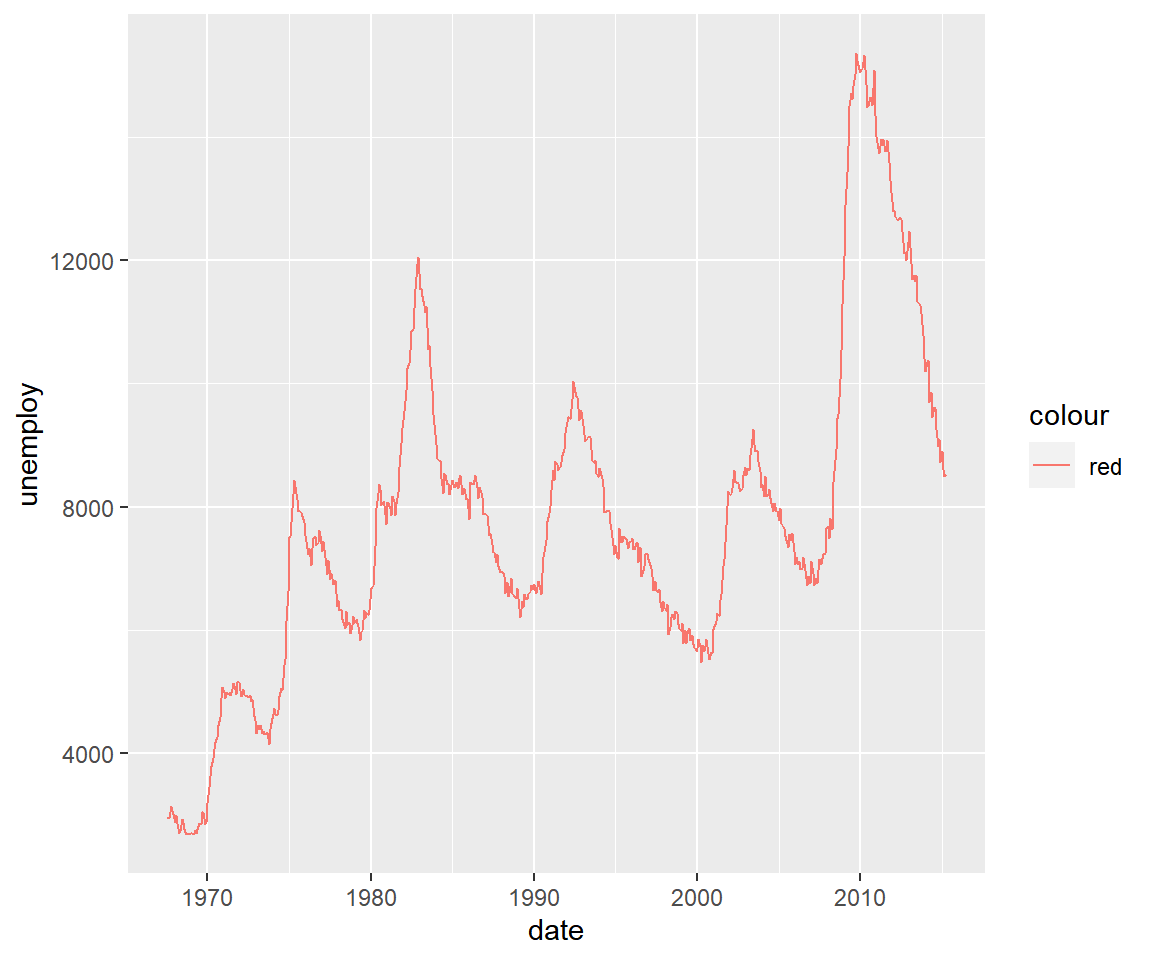#### Histogram

A histogram is a plot that can be used to examine the shape and spread of continuous data. It looks very similar to a bar graph and can be used to detect outliers and skewness in data. A histogram is created using the `bins` argument as shown below. The first input is the name of the continuous variable and the second is the name of the data set.

``qplot(mpg, data = mtcars, bins = 5)``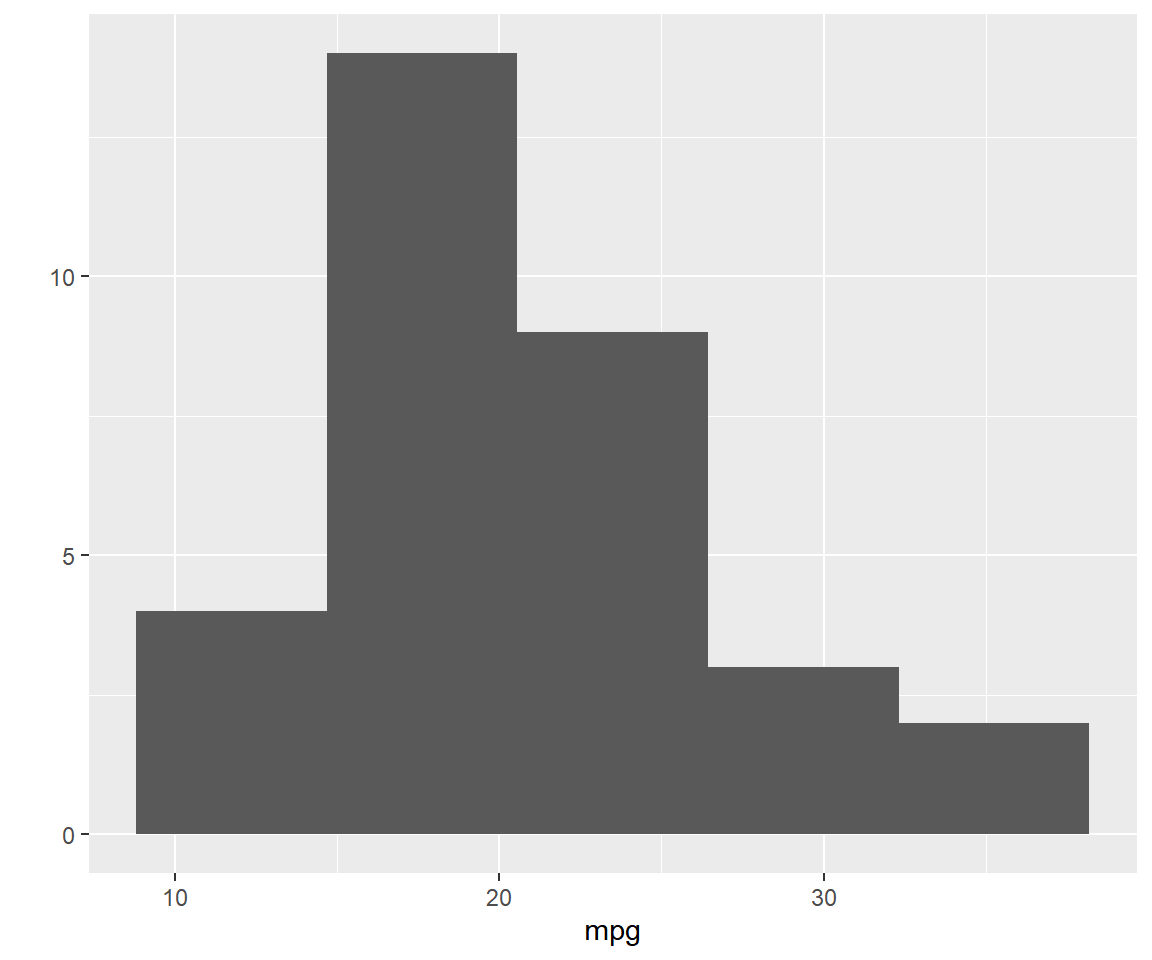### Summary

In this post, we learnt to quickly create plots using the `qplot()` function. While useful, it has limitations and can be used only to quickly visualize data.

### Up Next..

In the next post, we will create the same set of plots but using geoms.